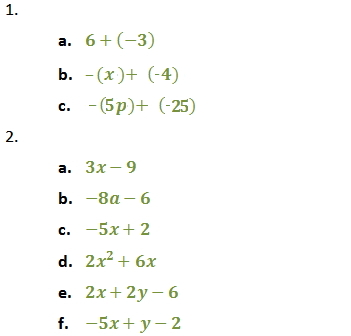Distributive Property

Distributive Property

a(b + c) = ab + ac

Parentheses can be removed by multiplying the coefficient to each term inside the parentheses.

Note: A negative sign outside parentheses can be understood as the coefficient -1. The distributive property may then be applied to remove the parentheses.

Example: -(4x + 3)

= -1(4x + 3)

= -4x - 3

Recall also that subtracting a number is the same as adding its negative.

Example:  7 - 4

= 7 + (-4)

Example:  6 - (-2)

= 6 + 2

Practice

1.  Rewrite each subtraction expression below as adding its opposite.

a)  6 - 3

b)  -x - 4

c)  -5p - 25

2.  Use the distributive property to remove parentheses.

a)  3(x - 3)

b)  -2(4a + 3)

c)  -(5x - 2)

d)  2x(x + 3)

e)  2(x + y - 3)

f)  -(5x - y + 2)